PHY294H - Lecture 2
Recommended work related to lecture 2:
Do problems: 22.24, 22.30, 22.48, 22.56
Go through concept questions: Chapter 23, questions 1-7

Example - uniform ring of charge

Consider a thin perfectly circular ring centered at the origin, with radius R, lying in the x-y plane and being uniformly charged with linear charge density. Find the force on a charge q placed at position,.

Solution

By symmetry, the x and y components of the force on the charge are zero. We are thus left with the task of calculating the z-component. The distance, r, from the charge to any point on the ring is given by,

 r2 = R2 + z2 (1)

If we defineto be the angle that the vector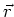makes with the z-axis, then,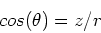(2)

The total force on the charge is then,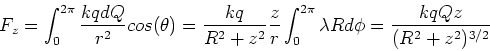(3)

where we usedThe electric field

The electric field is a very important concept in all of electromagnetism. At first it seems like it is the same as Coulomb's law. In fact it is. However it is a much better way of thinking about electrostatic forces.

Definition: Electric field

The electric field at a pointdue to a charge distribution is defined in terms of the force on a positive test charge, q, placed at position. The precise definition is,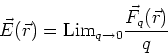(4)

We can think of the electric field as the force per unit positive charge at position. Notice that once we have found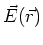, we can find the force on any charge, q, by using.

Superposition works for the electric field (Obviously!!)

From the definition of the electric field, we write Coulomb's law as,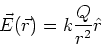(5)

That is we find the electric field due to one of the charges and then multiply,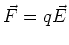, to find the force between the two charges, ie. Coulomb's law.

In a similar way, the electric field due to a distribution of discrete charges is given by,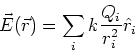(6)

This is the vector sum of the electric fields due to each of the point charges, Qi. When we have continuous distributions of charges, Eq. (6) becomes an integral as discussed in the last lecture.

Example- discrete charge distribution

Consider three charges located (and fixed) at (x,y) positions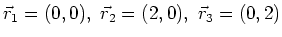(in meters) and having charges. Find the force acting on charge q3 due to the other two charges. What is the electric field at position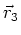due to charges q1 and q2?

Solution

We need to treat the force as a vector. In this problem the best way to treat the force is to resolve the Coulomb force between each pair of charges into its x-components and it y-components. The charges form an isosceles right-angle triangle, with charge q1 lying at the right-angle corner. The other angles in the triangle are 45o. The x-component of the force on charge q3 is given by,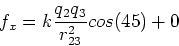(7)

The y-component of the force on charge q3 is given by,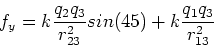(8)

The electric field at positiondue to charges q1 and q2 is,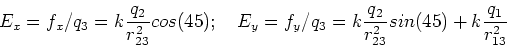(9)

Example - uniform ring of charge

Find the electric field for the thin charge ring discussed above.

Solution(10)

Electric dipole

An electric dipole consists of two charges of equal and opposite sign (-q,q), separated by distance L. As we shall see below, the electric dipole moment is the product p=qL, which measures the strength of the dipole. A vector direction is also assigned to the electric dipole moment, and this direction is chosen to be from the negative charge to the positive charge. Consider an electric dipole, centered at the origin and lying along the x-axis, with the positive charge at x-position, L/2. Find the electric field due to this dipole at any point in the x-y plane. Notice that this is the general solution as there is rotational symmetry about the x-axis.

At any point P located at (x,y) in the x-y plane we define r1 to be the distance from the positive charge to the point (x,y),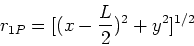(11)

r2P is the distance from the negative charge to the point (x,y),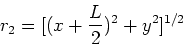(12)

The electric field at any point in the plane is then,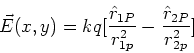(13)

Usually, the point P is a long way from the dipole, so we make the approximation that L<<r = (x2+y2)1/2. In that case a neat expression can be worked out, however it is easier once we have developed the idea of the electric potential. Here we shall analyse a special case, namely the case where x=0. In that case, we have,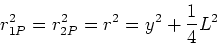(14)

The angle,, between the vector direction from the positive charge to point P obeys,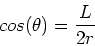(15)

The electric field at x=0 is then,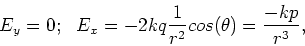(16)

Notice that Ex is proportional to p = qL and that it decays as 1/r3 which is a stronger decay than that of an isolated charge. p=qL is called the dipole moment and it controls the strength of dipole electric fields. The dipole moment is assigned a direction, which is a unit vector starting at the negative charge in the dipole and going toward the positive charge.

Electric field lines

1. At each point along an electric field line, the force on a positive test charge is in a direction tangent to the field line at that point.

2. The density of lines at any point in space is proportional to the magnitude of the electric field at that point.

3. Electric field lines begin and/or end at charges, or they continue off to infinity. i.e. they do not begin or end in free space.

4. Electric field lines do not cross.

Very important special case: conductors

1. If there is no current flowing, then the electric field is zero,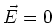, inside a conductor.

2. If there is no current flowing, then at the surface of a conductor, the electric field is normal to the surface of the conductor.